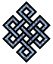fail2ban bad ip database: ip 125.71.211.10

| ip database | live view | stats | report | help | api key:

 ip: 125.71.211.10 hostname: 125.71.211.10 country:[CN] China first reported: 21.11.2018 03:53.10 GMT+0200 last reported: 27.03.2019 00:53.06 GMT+0200 time period: 125d 20h 59m 56s total reports: 12 reported by: 2 host(s) filter(s): ssh (7) ssh (5) tor exit node no badips.com db Lookupport scan of '125.71.211.10':

[-hide]
```# Nmap 6.40 scan initiated Wed Nov 21 03:54:02 2018 as: /usr/bin/nmap -sU -sS -O 125.71.211.10
Nmap scan report for 125.71.211.10
Host is up (0.25s latency).
Not shown: 999 open|filtered ports, 987 filtered ports
PORT      STATE  SERVICE
80/tcp    open   http
443/tcp   open   https
1935/tcp  open   rtmp
3306/tcp  open   mysql
8011/tcp  closed unknown
8081/tcp  open   blackice-icecap
8200/tcp  closed trivnet1
8443/tcp  open   https-alt
9081/tcp  open   unknown
10001/tcp open   scp-config
20000/tcp open   dnp
30000/tcp closed unknown
500/udp   open   isakmp
No exact OS matches for host (If you know what OS is running on it, see http://nmap.org/submit/ ).
TCP/IP fingerprint:
OS:SCAN(V=6.40%E=4%D=11/21%OT=80%CT=8011%CU=%PV=N%G=Y%TM=5BF4C91F%P=x86_64-
OS:pc-linux-gnu)SEQ(SP=107%GCD=1%ISR=10B%TI=Z%CI=I%TS=8)OPS(O1=M5B4ST11NW7%
OS:O2=M5B4ST11NW7%O3=M5B4NNT11NW7%O4=M5B4ST11NW7%O5=M5B4ST11NW7%O6=M5B4ST11
OS:)WIN(W1=7120%W2=7120%W3=7120%W4=7120%W5=7120%W6=7120)ECN(R=Y%DF=Y%TG=40%
OS:W=7210%O=M5B4NNSNW7%CC=Y%Q=)T1(R=Y%DF=Y%TG=40%S=O%A=S+%F=AS%RD=0%Q=)T2(R
OS:=N)T3(R=N)T4(R=Y%DF=Y%TG=40%W=0%S=A%A=Z%F=R%O=%RD=0%Q=)T5(R=Y%DF=Y%TG=80
OS:%W=0%S=Z%A=S+%F=AR%O=%RD=0%Q=)T6(R=Y%DF=Y%TG=80%W=0%S=A%A=O%F=R%O=%RD=0%
OS:Q=)T7(R=N)U1(R=N)IE(R=N)

OS detection performed. Please report any incorrect results at http://nmap.org/submit/ .
# Nmap done at Wed Nov 21 03:55:27 2018 -- 1 IP address (1 host up) scanned in 86.11 seconds
```
```Σ = 88 | Δt = 0.0045289993286133s
```